### 10 Math Problem Solving Websites to get instant answers

are you looking for free Math Problem Solving Websites with step-by-step explanations? well, you are in the right place. This good math solving websites from algebra, trigonometry, matrices, to calculus. also, you can put your own math problem to get help from experts and teachers. you can also try to take a photo of any math problems and solve them using the math solver apps. there are math tests and quizzes teachers could use to develop exams.

in this article, we compiled 10 websites that solve math problems with steps.

## Microsoft math solver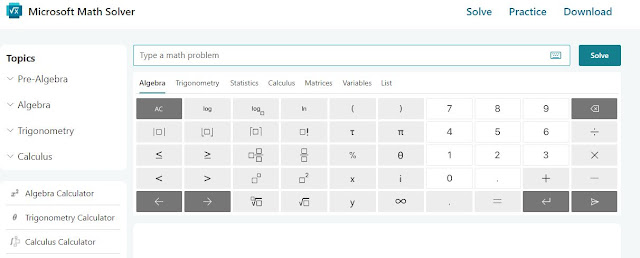the best site to solve math problems which give you detailed solutions to any math question, From algebra and quadratic equations to statistics and trigonometry, step by step. It also helps to get an Instantly graph of any math equations. in addition to relevant practice examples, and online video tutorials to figure out math problems.

## Mathway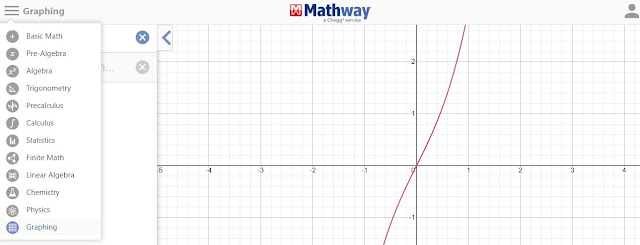mathway best math solver website with a lot of tools to answer any math question from Pre-Algebra, Statistics to chemistry. all you have to do is just put in math problems and get answers with steps. It also provides supporting materials such as interactive graphs, a limit calculator, and solve calculus problems.

## QuickMath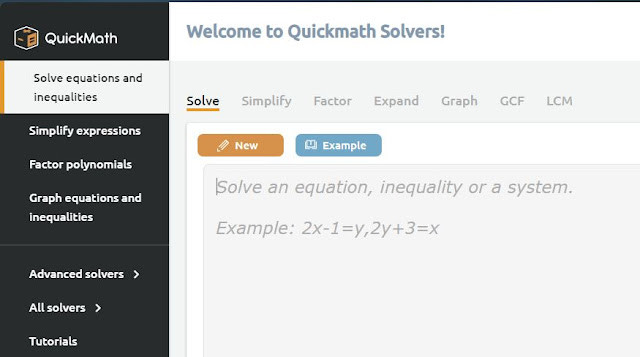quickmath is an online maths solving website that deals with all math problems types related to matrices, factor polynomials, and Graphing equations with a detailed explanation until you reach the full answers. in addition, it has tutorials on all math topics even how to use quickmath to simplify expressions.

## Symbolab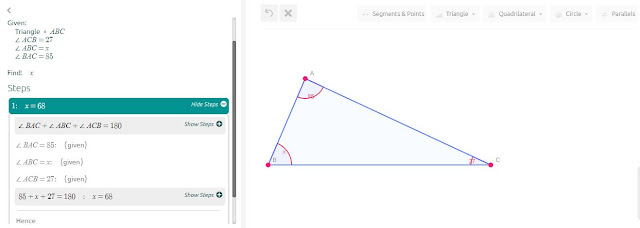symbolab is one of the websites that give you answers to math problems relevant to algebraic, calculus, and geometry, covering topics from elementary school to college. with his new geometry solver feature enables you to calculate angles to any triangle, find radius to circle, and a lot of shapes in detailed steps.

## wolfram alpha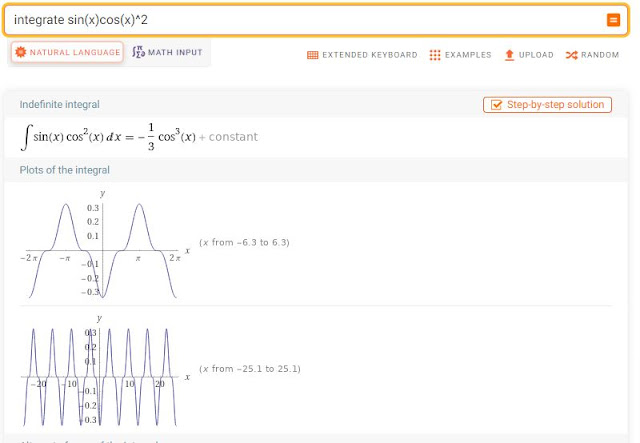wolfram alpha is a website that shows you the steps to solve math problems from elementary basic maths to advanced ones. you can plot any statistical data into pie charts with different inputs Descriptive. this math solver site allows you to scan math problems and obtain Step-by-Step Solutions in all maths types.

## Cymathcymath website that solves math equations with fully described steps with the ability to check the answers for a good understanding of the whole solution. also, there are a lot of practice examples from basic algebra to calculus.

## Math10math10, a website for math answers and steps for any algebra, Matrix Solvers, or calculus-related problem. with tutorial articles on any mathematics topics. also, it has an interactive maths test for all ages from first grade to Intermediate.

## Tutorbintutorbin, a website that will do math problems for you from matrix algebra, and calculus to quadratic equations. When it comes to tackling Algebra issues, this is a student's best buddy.it improves students' grades by solving any math homework in full detail steps.

## Chegg math solverChegg, is the best math solver website to understand equations step by step by Breaking down the important steps into smaller substeps to show each part of the solution.in addition, you can take a photo of your maths equations to solve them instantly.

## Brainlybrainly, a website to put in math problems and get answers in a detailed explanation from experts. just snap math word problems and the community will solve them for you. also, there are textbooks that cover maths problems for all student levels.